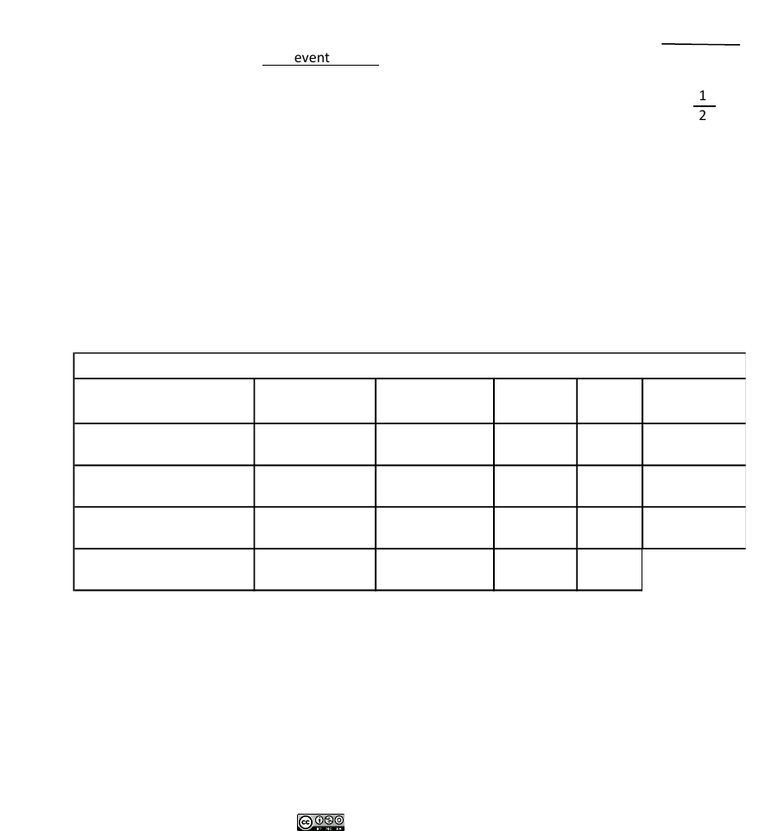Study Guides (400,000)
US (230,000)
Rutgers (3,000)
8:27 (30)
M.Verzi (10)
Final

# 01:447:384 Chapter Notes - Chapter 1: Mutual Exclusivity, Gregor Mendel, Crop YieldExam

Department
Genetic
Course Code
01:447:384
Professor
M.Verzi
Study Guide
Final

This preview shows half of the first page. to view the full 3 pages of the document.Understanding the Application of Probability to Genetics
Dr. Valerie Schawaroch BIO 3015 Principles of Genetics Spring 2018
“Mathematics is the language of science.” What does this statement mean? Well in the case of Classical or
Transmission genetics, the inheritance patterns for the resulting offspring of a cross can be predicted using
probability theory. In this lab you will study (1) the probability of independent events occurring simultaneously and
(2) the probability of either one or the other of two mutually exclusive events occurring.
Probability is the likelihood of ‘something’ occurring. This likelihood gives an ability to predict. Predicting events
based on a set of circumstance is the function of science. Gregor Mendel employed the scientific method and use
probability theory to predict outcomes of mating or crosses. He did this to help the general public who were mostly
farmers. Instead of trial and error farmers could use these methods to mate certain plants (or livestock) to improve
crop yield or milk quality, for example. Mendel hypothesized that the inheritance of factors (alleles) for traits
(genes) to be random and independent. Even though events are random they still can be predicted. For example, the
odds of winning a lottery.
Predicting Random Events
There are two sides to a coin - a heads side and a tails side. Heads or tails is considered an event. If you want to
determine the likelihood of obtaining heads when you toss a coin you have to divide the event (heads) by the total
Here is a general formula you can use:
Use this formula for a different example rolling one dice (this is a cube [has six sides] each side has a single dot [=1]
or multiple dots to represent one of the numbers 2 through 6).
You roll one dice and one side is face up. What is the probability that the face up side is 3? ________
Probability Rules
Students working in pairs should toss two coins simultaneously 24 times. Record your observations in Table 1
below for heads on both coins (HH), one head and one tail (which can occur in either order HT or TH), and tails on
both coins. Note each coin is an independent event because the side that is face up on the first coin does not affect
the side that faces up on the other coin.
To calculate the values for the expected column the multiplication (or product) rule and the addition (or sum) needs
to be applied.
The multiplication rule: with respect to independent events, when one event and a second event occur at the same
time, you multiply their two probabilities.
The sum rule: are mutually exclusive events, either…or situations. In such cases the probabilities of each event are
Probability of event
=
event
total possibilities
=
1
2
=
Example:
Table 1
Event Combinations
Observations (O)
Expected
(E)
O - E
percent
difference